# Angles

 1 Introduction to Angles 2 Types of Angles and their Properties 3 How to measure an Angle? 4 Thinking out of the Box! 5 An Angle and its Reflex Angle 6 How to construct Angles? 7 Interior and Exterior Angles 8 Common Mistakes 9 Complementary and Supplementary Angles 10 Some more types of Angles 11 What is Transversal? 12 Exterior Angle Property of a Triangle 13 Angle types based on Rotation 14 Important Notes on Angles 15 Solved Examples on Angles 16 Practice Questions on Angles 17 Important Topics of Angles 18 Maths Olympiad Sample Papers 19 Frequently Asked Questions (FAQs) 20 FREE Worksheets of Natural Numbers

We at Cuemath believe that Math is a life skill. Our Math Experts focus on the “Why” behind the “What.” Students can explore from a huge range of interactive worksheets, visuals, simulations, practice tests, and more to understand a concept in depth.

Book a FREE trial class today! and experience Cuemath’s LIVE Online Class with your child.

## Introduction to Angles

When two lines intersect at a point, the measure of the “opening” between these two lines is called an “Angle”. It is denoted using the symbol $${\angle}$$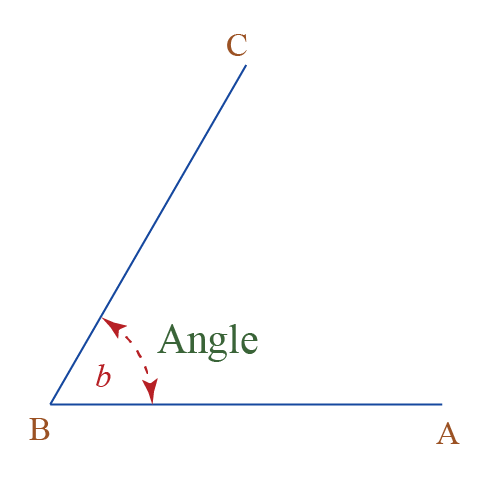Angles are usually measured in degrees and denoted by $${\circ}$$ (the degree symbol), which is a measure of circularity or rotation.

Angles are a part of our day to day life. Engineers and architects use angles for the design of roads, buildings, and sporting facilities.In the above image, we can see a surveyor using a Theodolite at a construction site to measure the angle.

Let’s see, how many of you like sports!

Have you ever watched a game of football?

Did you observe where the players take the corner kick from? Well, the point at which the lines intersect is what forms an angle!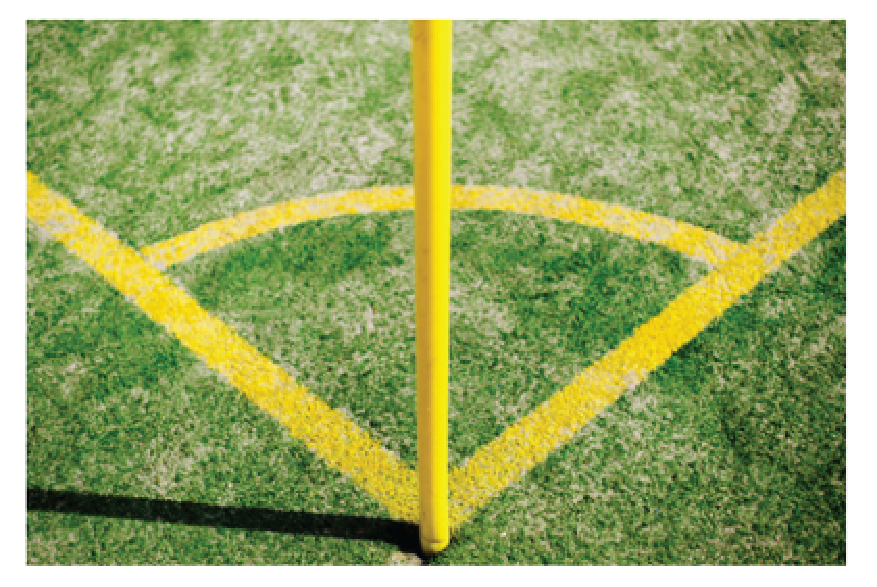### Parts of an Angle

In plane geometry, an angle is formed when two rays originate from the same endpoint. There are two parts of the angle-

1. two rays, which are called the sides of the angle
2. the vertex of the angle, which has a common endpoint that is shared by the two rays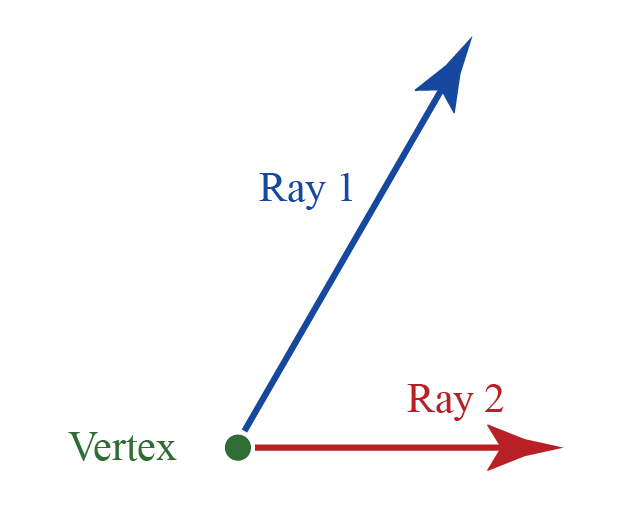### Angles and Degrees

An angle is measured in degrees. There are 360 degrees in one Full Rotation (one complete circle around).

### Size of an Angle

The best way to measure the size of an angle is to use a protractor. The standard size of a protractor is $${180^\circ}$$.

There are two sets of numbers on a protractor:

• one in a clockwise direction
• another in an anti-clockwise direction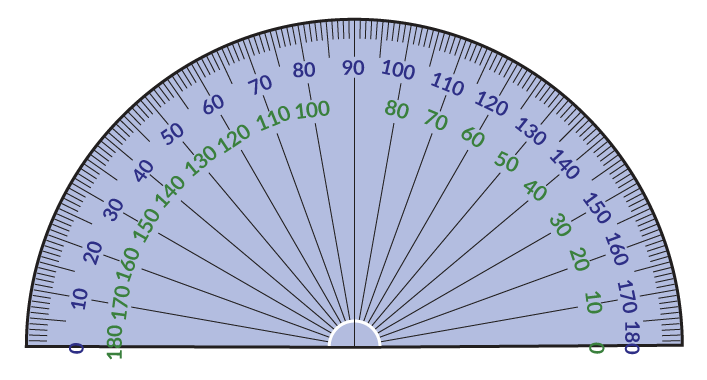## Types of Angles and their Properties

Type of Angle Description Example

1.

Acute Angle

An angle that is less than $${90^\circ}$$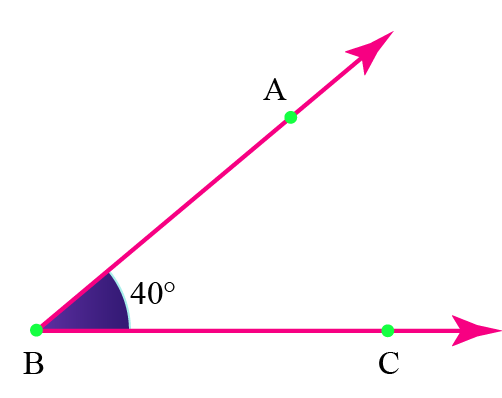2.

Right Angle

An angle that is exactly $${90^\circ}$$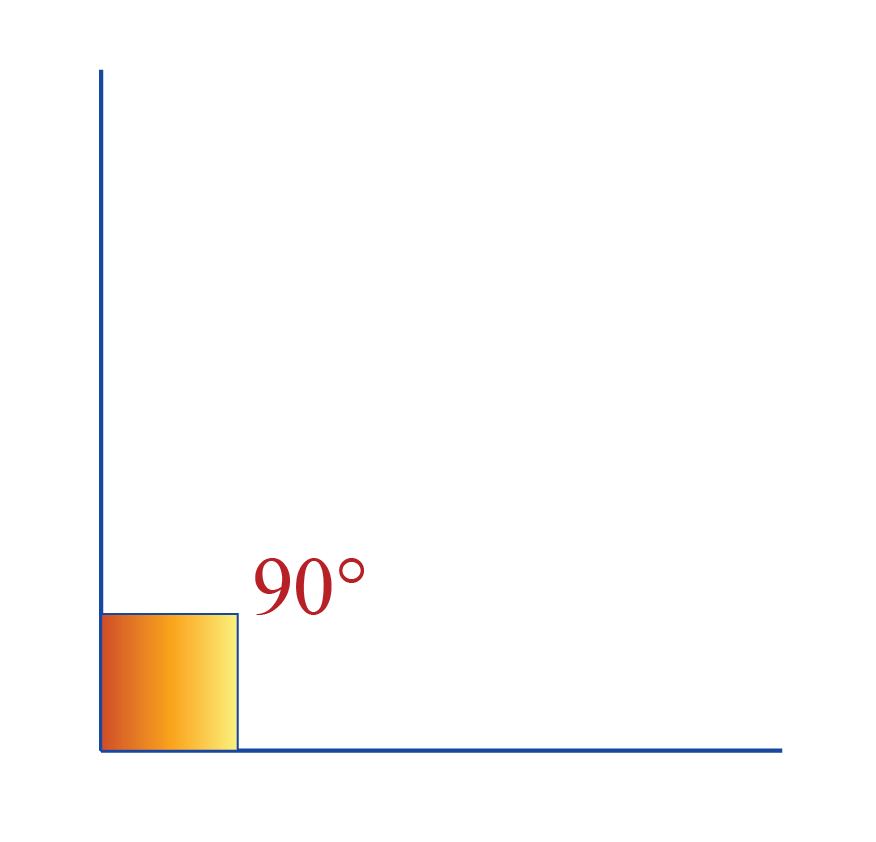3.

Obtuse Angle

An angle that is greater than $${90^\circ}$$ and less than $${180^\circ}$$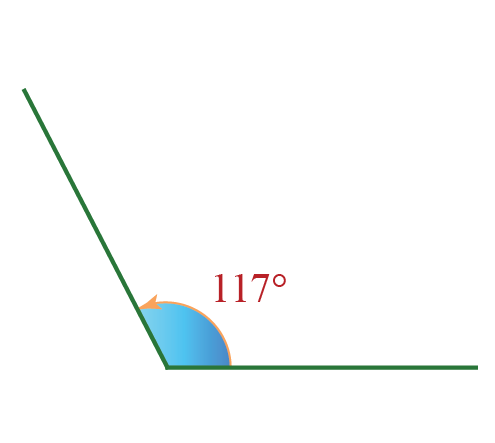4.

Straight Angle

An angle that is exactly $${180^\circ}$$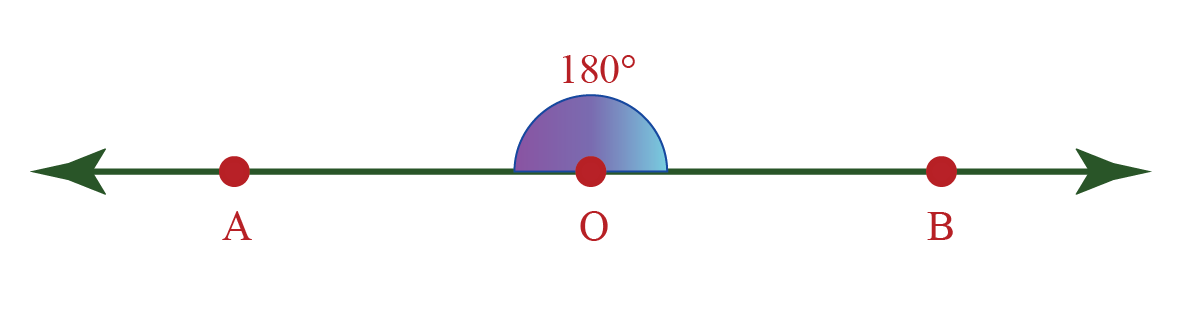5.

Reflex Angle

An angle that is greater than $${180^\circ}$$ and less than $${360^\circ}$$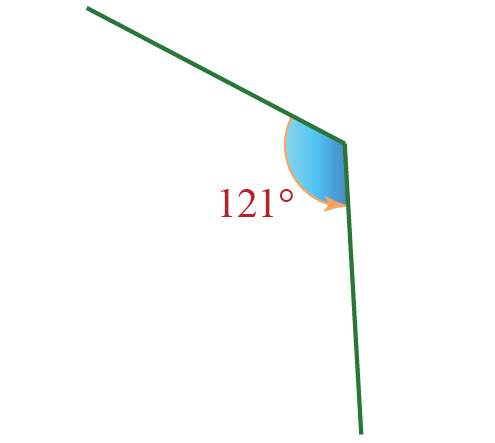6.

Complete Angle

An angle that is exactly $${360^\circ}$$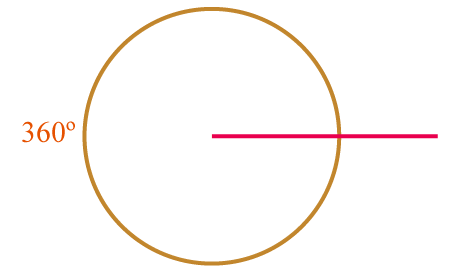### Acute Angle

An acute angle is an angle whose measure is greater than $${0^\circ}$$ and less than $${90^\circ}$$.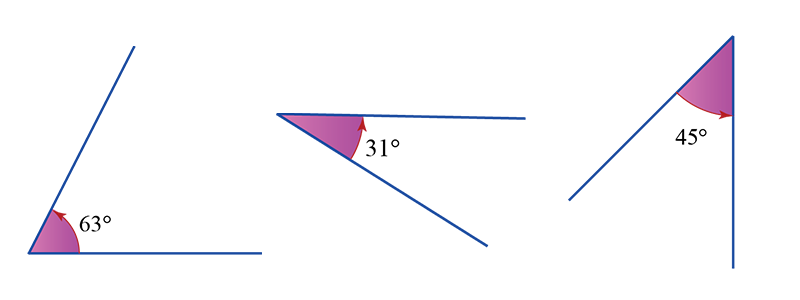### Right Angle

An angle that measures exactly $${90^\circ}$$ is called a right angle.

It is generally formed when two lines are perpendicular to each other.### Obtuse Angle

An obtuse angle is an angle whose measure is greater than $${90^\circ}$$ and less than $${180^\circ}$$.### Straight Angle

The angle formed by a straight line is called a straight angle.

It is one-half of the whole turn of a circle. The measure of the straight angle is $${180^\circ}$$.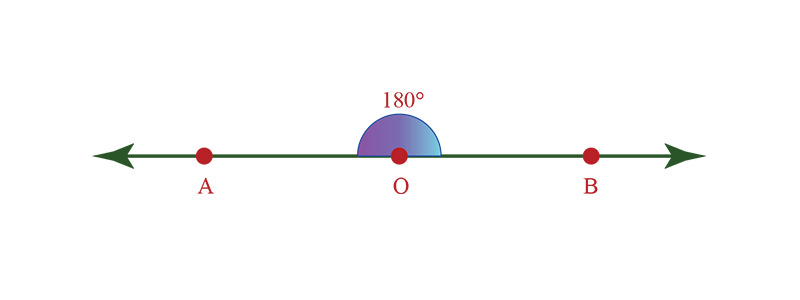### Reflex Angle

A reflex angle is an angle whose measure is greater than $${180^\circ}$$ but less than $${360^\circ}$$### Complete Angle

An angle whose measure is equal to $${360^\circ}$$ is called a complete angle.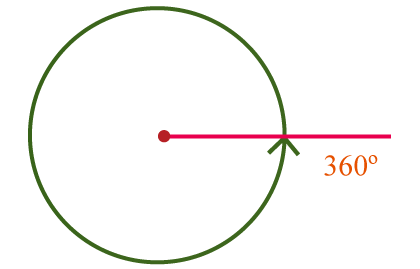In this simulation, you can drag the green line around the circle to see the type of angle that is formed between the two lines.

Observe the name of the angle formed at different degrees.

## How to measure an Angle?

We use protractors to measure angles.

See the image below. We can see $${\angle AOB}$$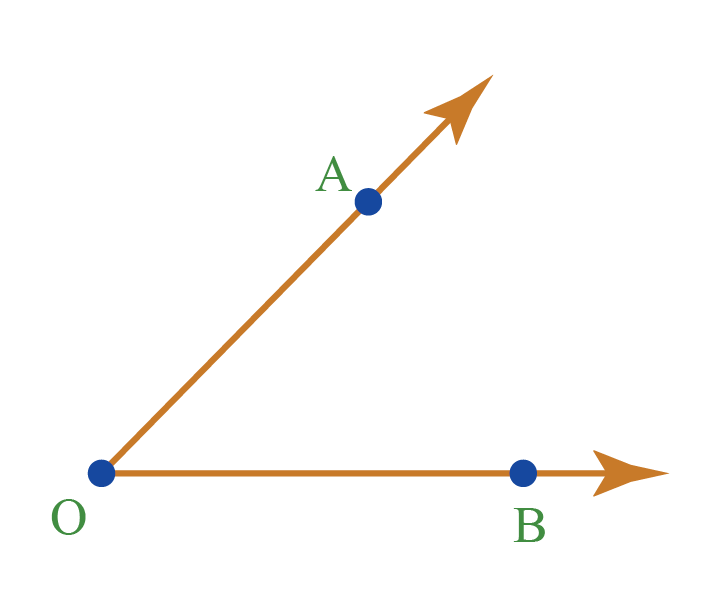Let’s try and see if we can find out what type of angle is $${\angle AOB}$$

Doesn't it look like an acute angle? This means that its measure is greater than $${0^\circ}$$ and less than $${90^\circ}$$.

Now let’s try to find its exact measure with the help of a protractor

Steps to measure $${\angle AOB}$$

### Step 1

Align the protractor with the ray OB as shown below.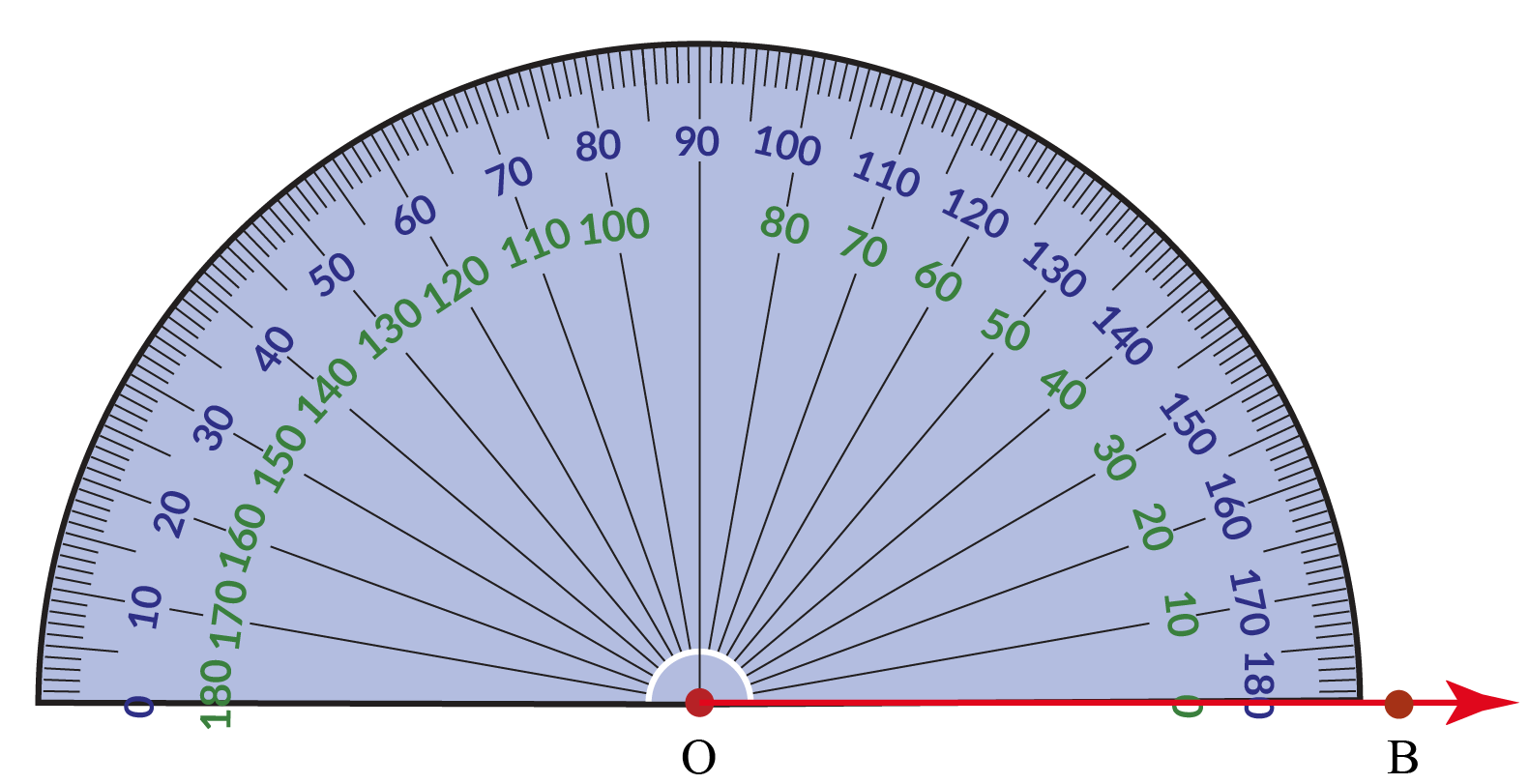Start reading from the $${0^\circ}$$ mark on the bottom-right of the protractor.

### Step 2

The number on the protractor that coincides with the second ray is the measure of the angle.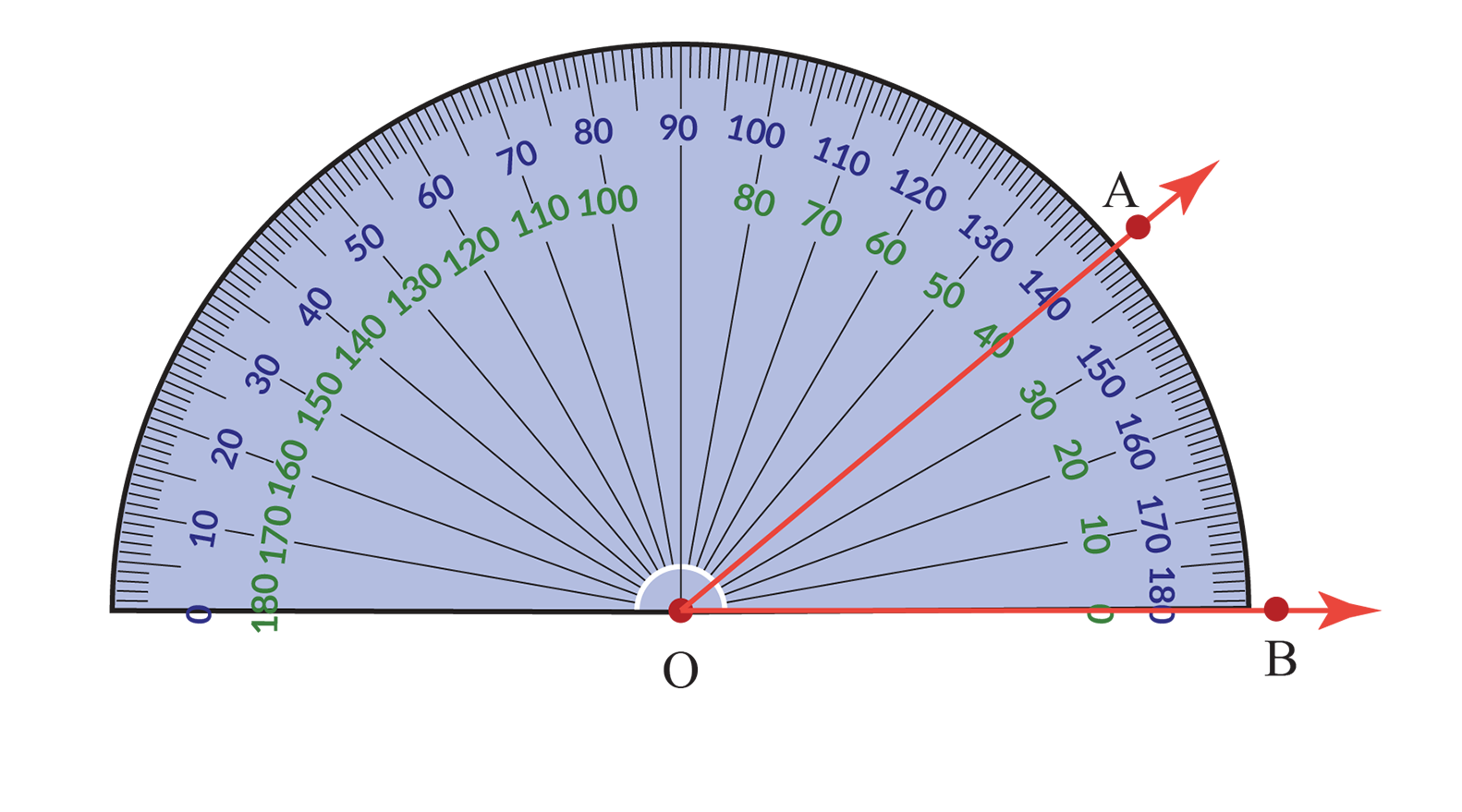### Step 3

Measure the angle using the number on the "lower arc" of the protractor.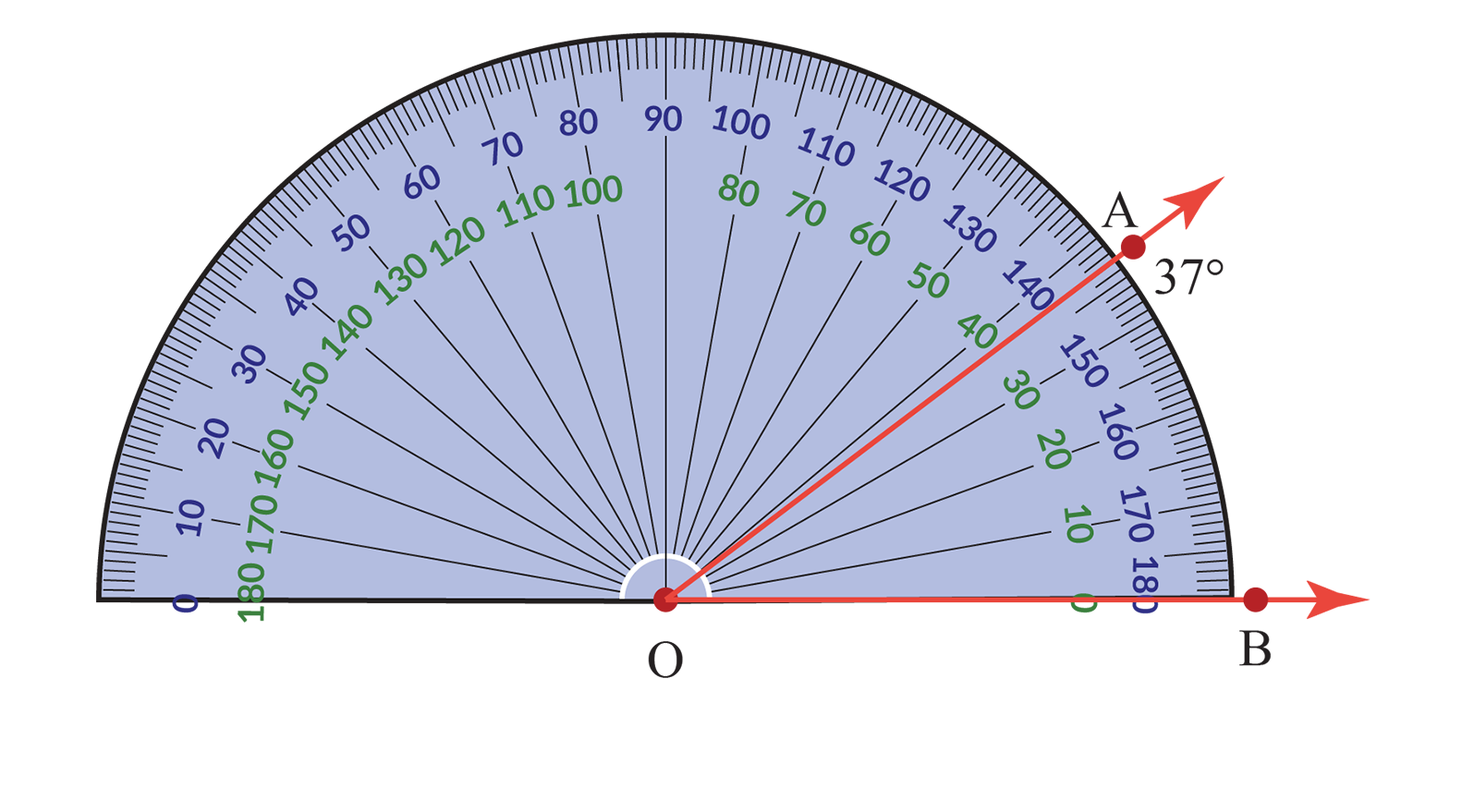\begin{align} \angle {AOB} = 37^\circ\end{align}

Next, let us try to measure this angle $${\angle AOC}$$.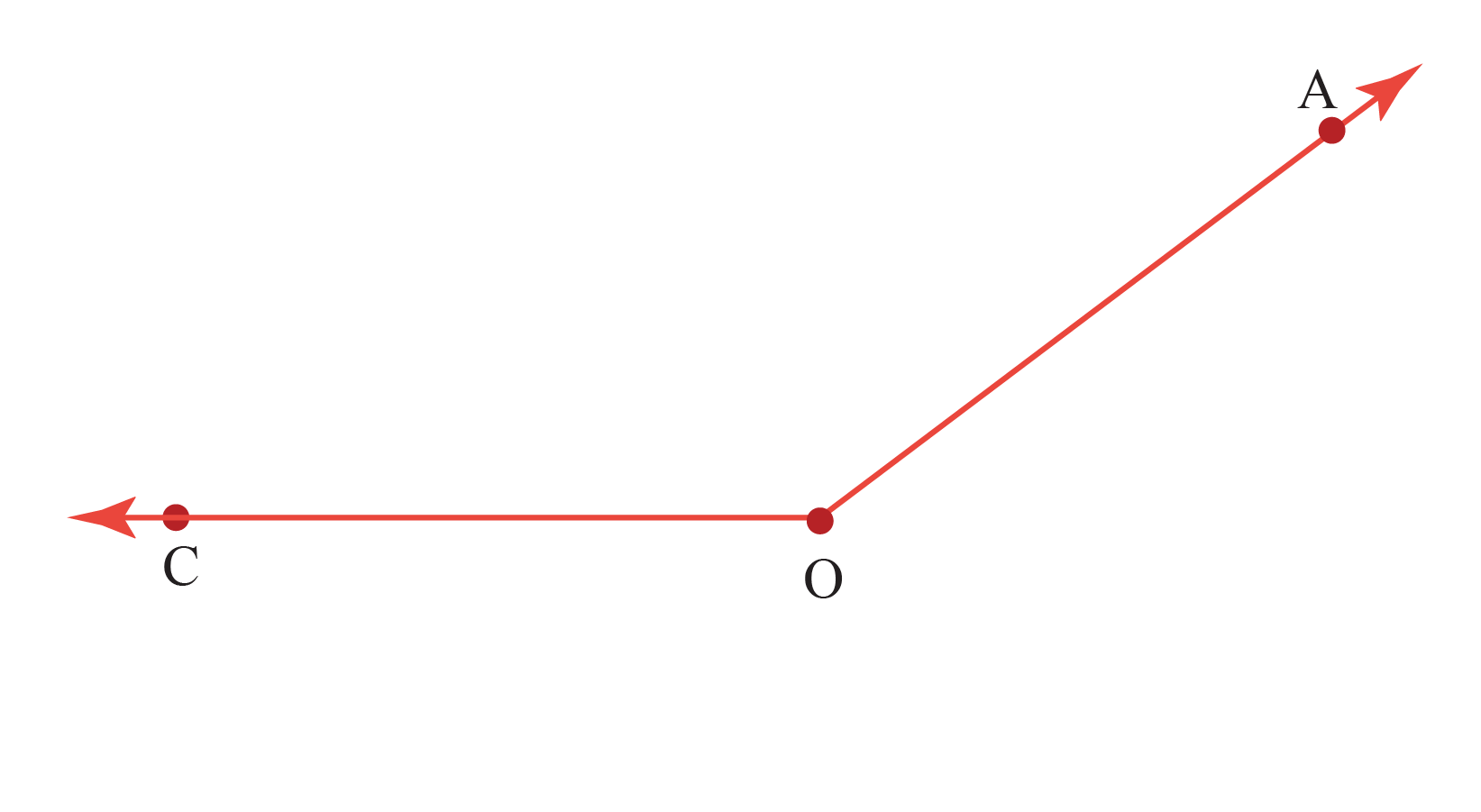### Step 1

Measure the angle from the $${0^\circ}$$ mark on the bottom-left.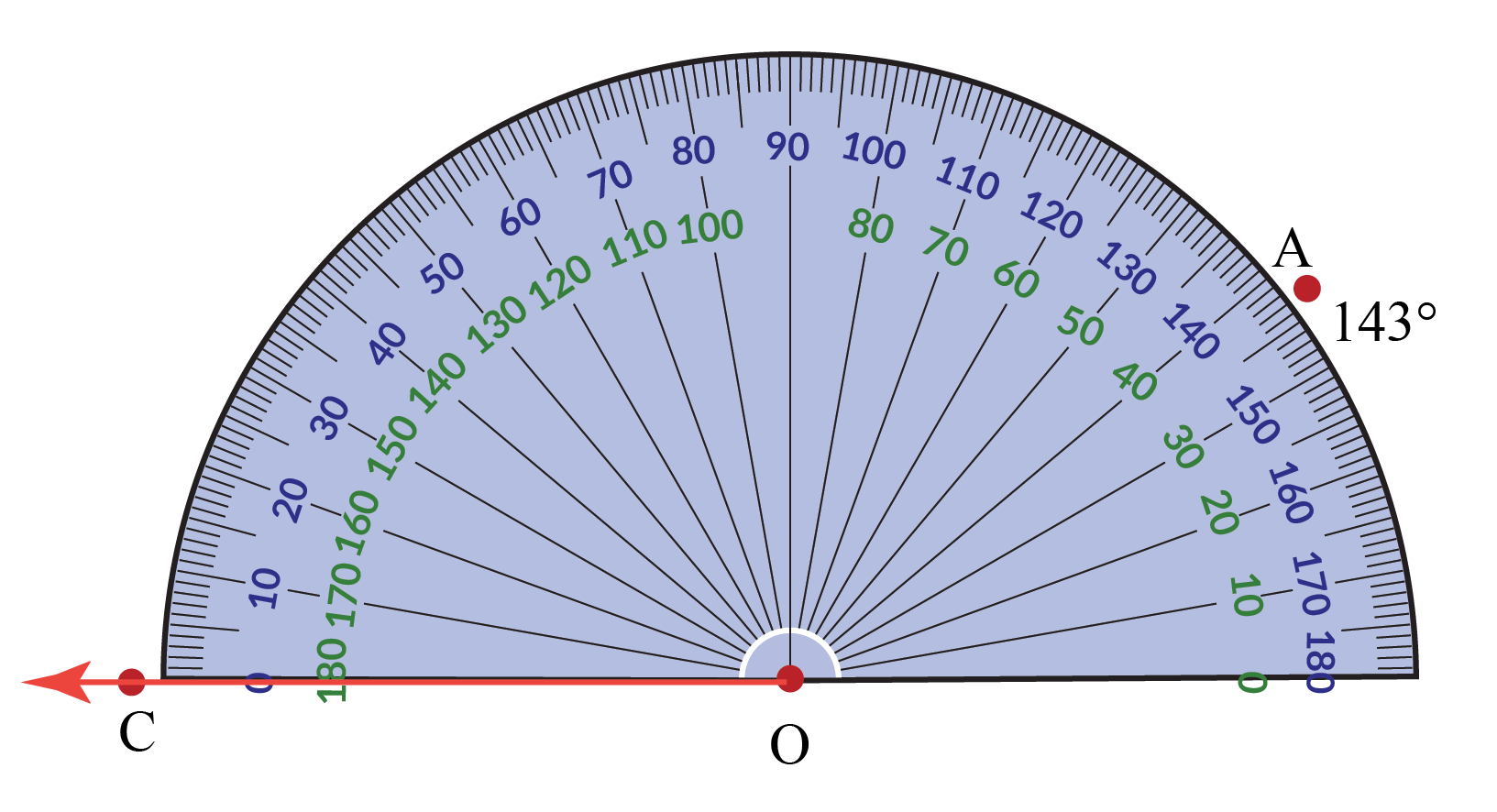### Step 2

The number on the "top arc" of the protractor that coincides with OA is the measure of $${\angle AOC}$$.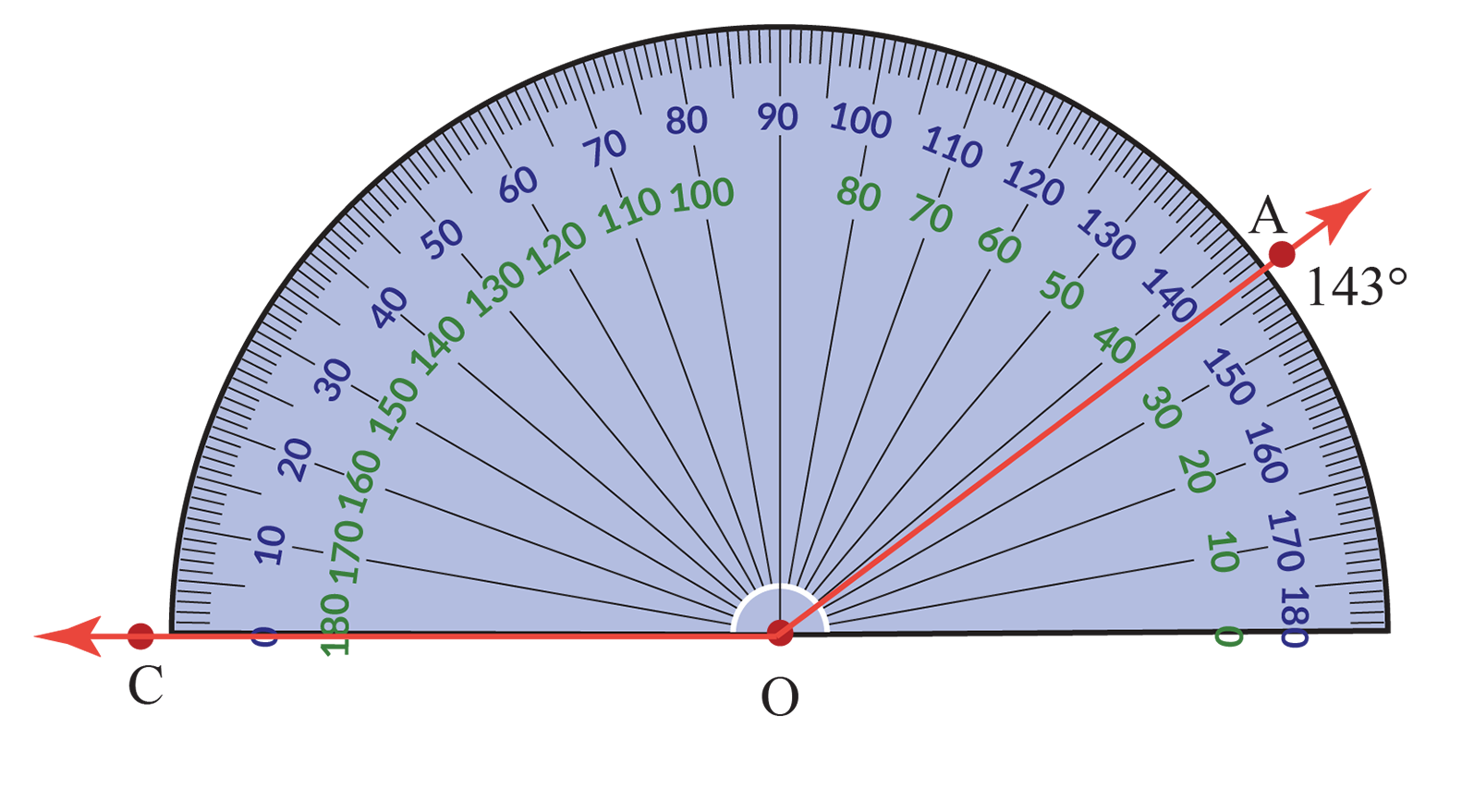\begin{align} \angle {AOC} = 143^\circ\end{align}

You can try measuring various angles in this simulation by following a few simple steps.

1. Enter the degree value that you want to measure on the protractor.
2. Now using the blue line, drag it along the protractor to measure the degree.
3. A right or wrong answer message will appear near the degree you entered to check your answer.Think Tank
1. Is $${30^\circ}$$ the same as $${-30^\circ}$$?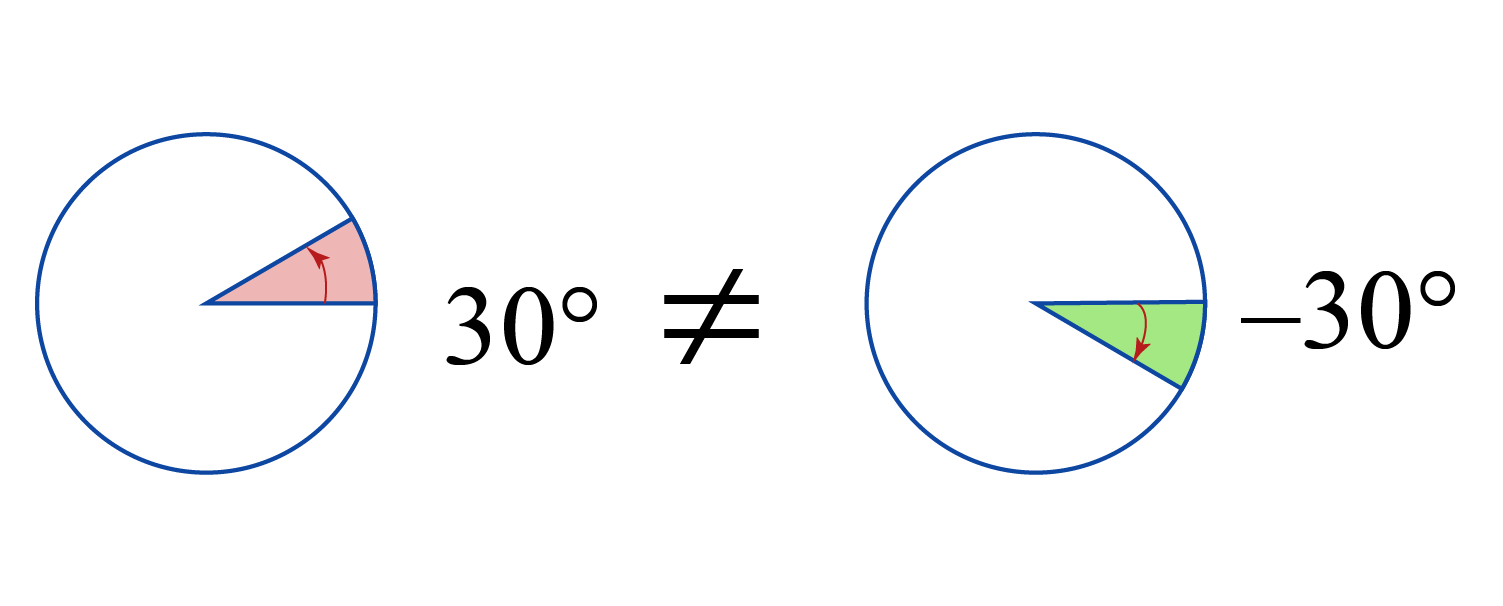## An Angle and its Reflex Angle

An angle is the "space" between two rays meeting at a common endpoint.

Let’s take the letters A,B, and O to name the angle.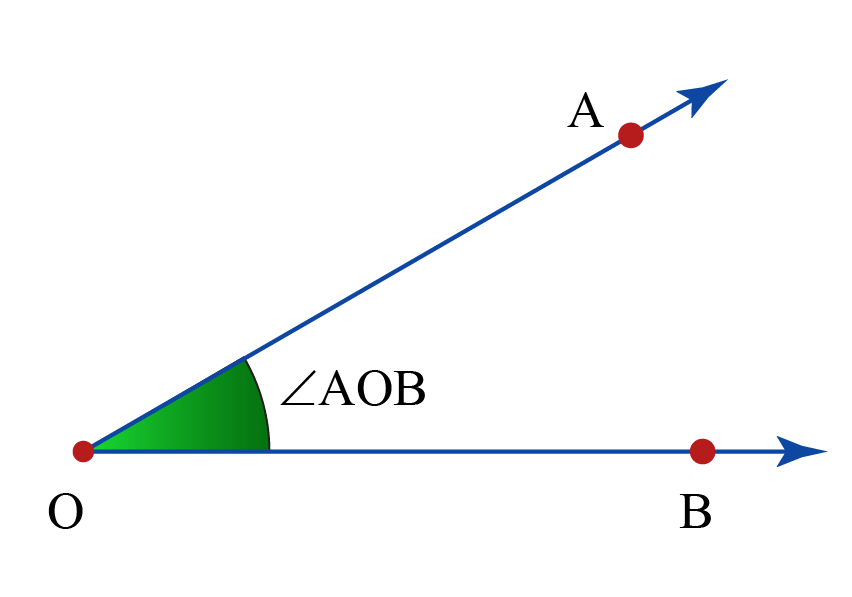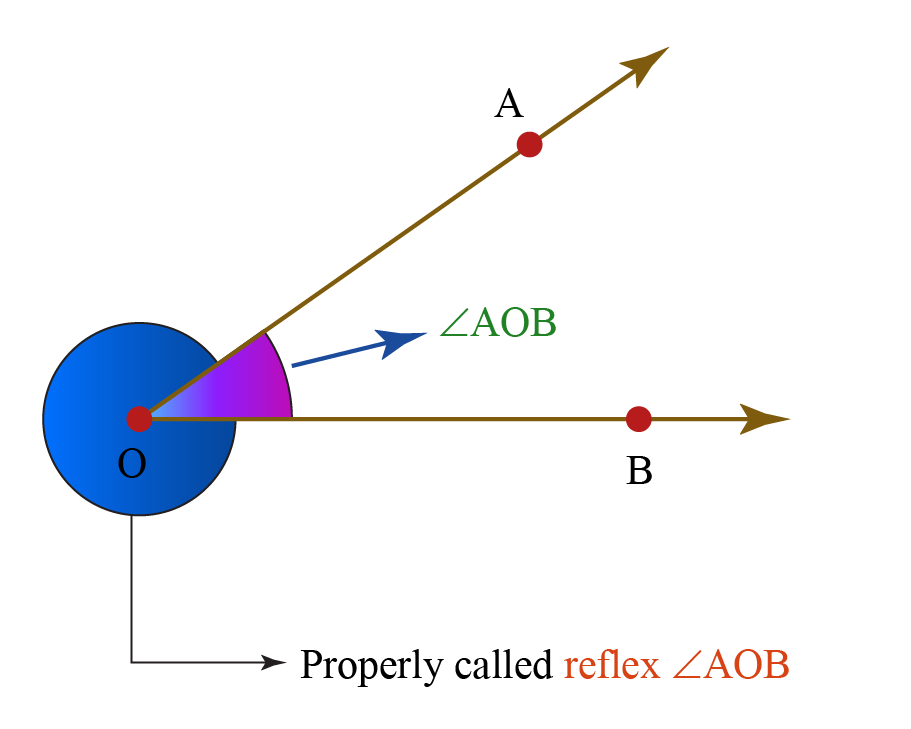The larger angle is a Reflex Angle, but the smaller angle is an Acute Angle

It is to avoid this particular ambiguity that we refer to the angle marked above (with the dotted line) as reflex $${\angle AOB}$$.

Note: any $${\angle AOB}$$ and its reflex $${\angle AOB}$$ sum to $${360^\circ}$$

This property of angle and its reflex can be used to measure (and construct) angles that measure more than $${180^\circ}$$ and less than $${360^\circ}$$.

## How to construct Angles?

We use a protractor to construct angles.

Let’s draw a $${50^\circ}$$ angle.

### Step 1

First, draw a ray OB and align the protractor with OB as shown.### Step 2

Place a point above the marking on the protractor that corresponds to $${50^\circ}$$.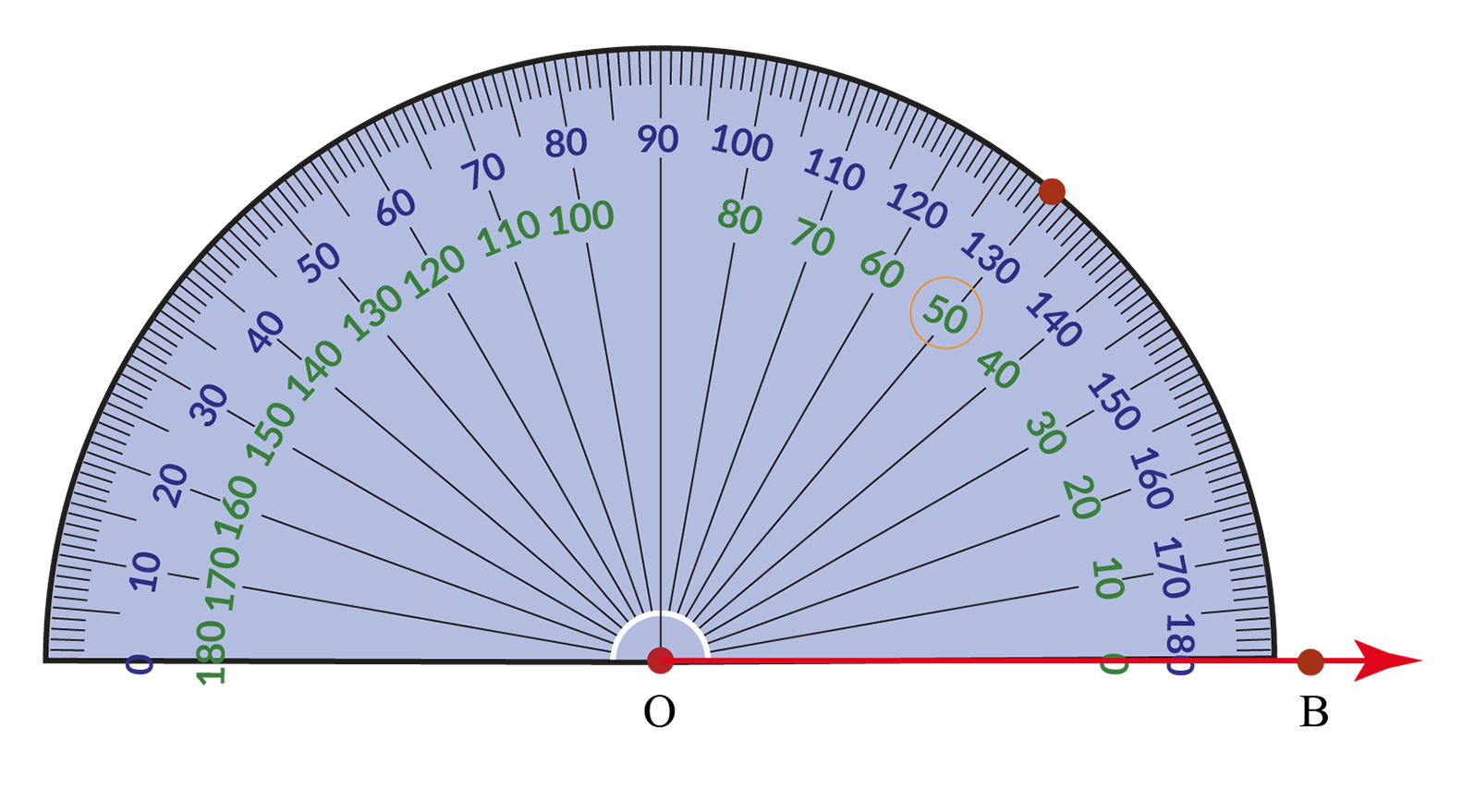### Step 3

Remove the protractor and draw a ray beginning at O that passes through this point.

$${\angle AOB}$$ is the required angle.

That is $${\angle AOB}$$ = $${50^\circ}$$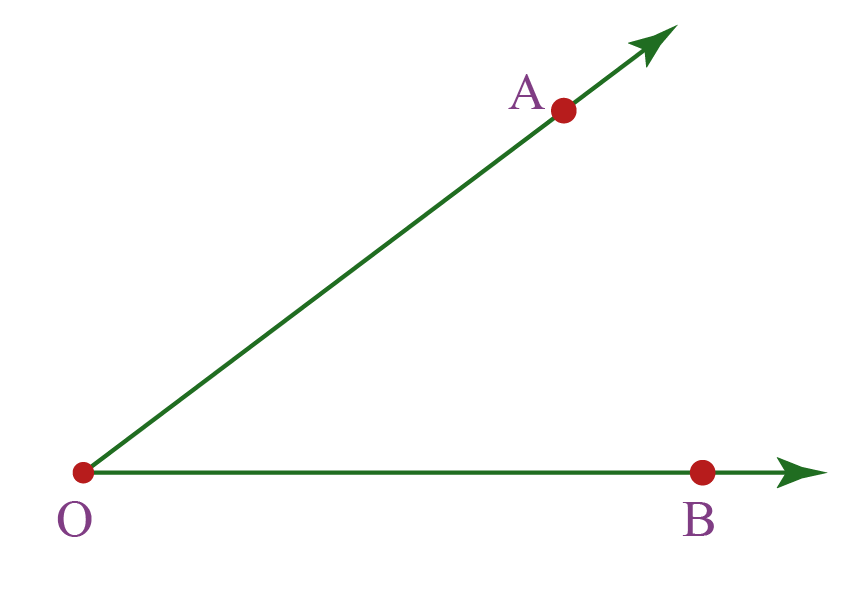### Step 4

If the ray extends in the other direction, we measure the angle from the $${0^\circ}$$ mark on the bottom-left.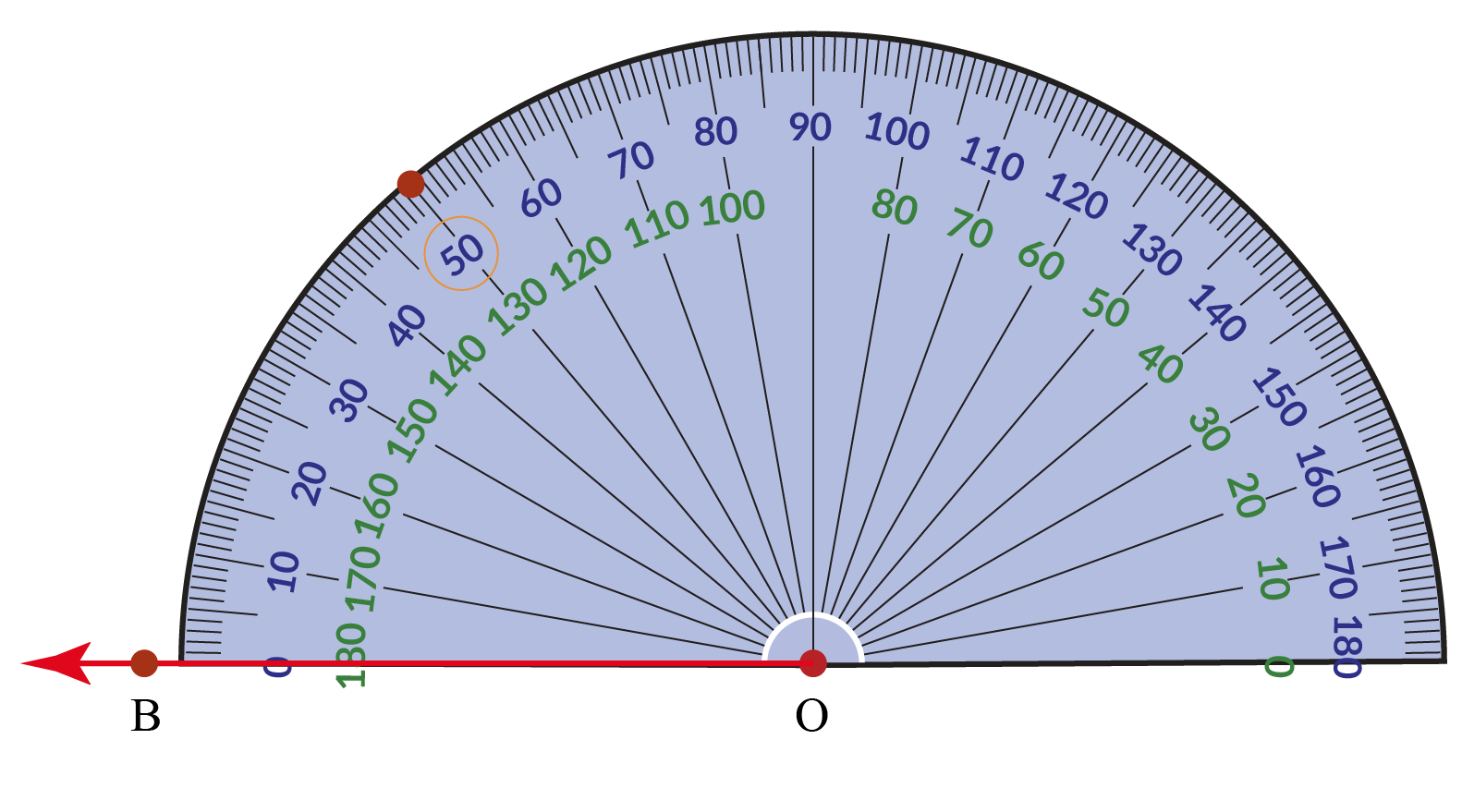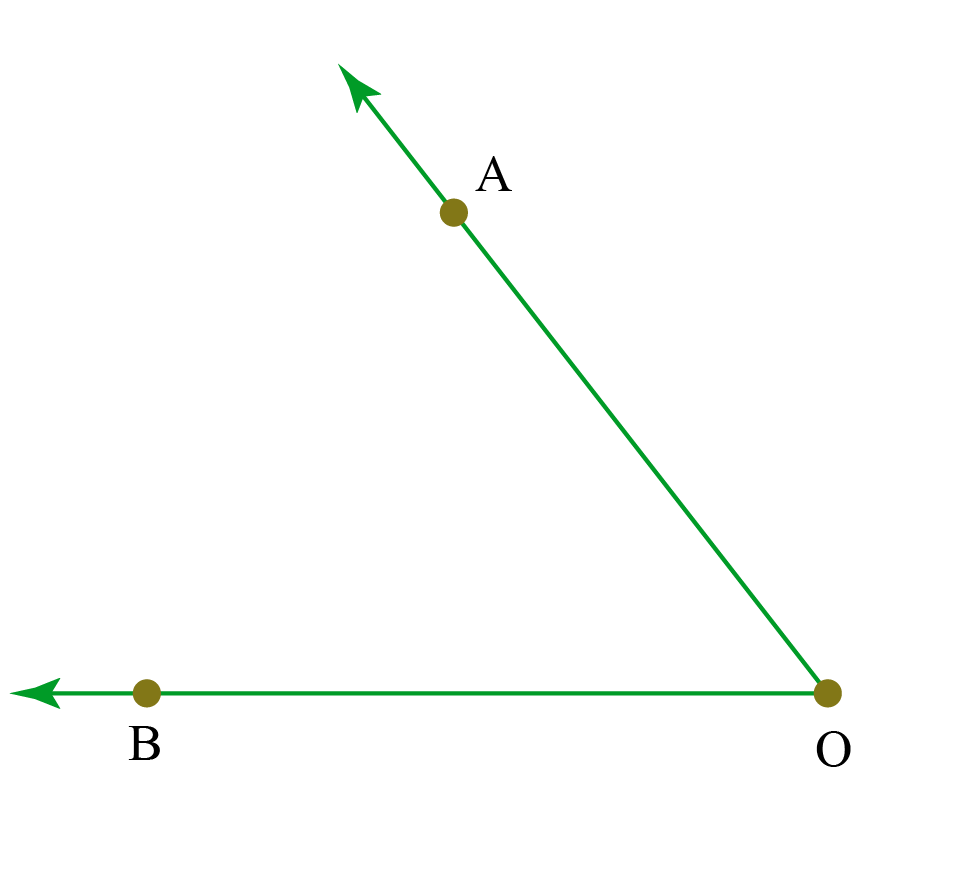The above image shows how to draw a $${50^\circ}$$ angle when the ray is pointing in another direction.Tips and Tricks
1. Mistake: Complementary angles have to be next to each other

Fact: When the sum of two angles is $${90^\circ}$$, the angles are called complementary angles. These angles need not be necessarily next to each other as long as their sum is $${90^\circ}$$

2. Mistake: Any angles next to each other are adjacent angles.

Fact: For angles to be adjacent, they have to share a common side and vertex.

## Interior and Exterior Angles

### Interior of an Angle

Interior angle is an angle inside the shape. The area between the rays that makes up an angle, and extending away from the vertex to infinity. The sum of the interior angles of a triangle is always 180 degree.

### Exterior of an Angle

The exterior angle is the angle between any side of a shape, and a line extended from the next side. The sum of an exterior angle and its adjacent interior angle is also 180 degree.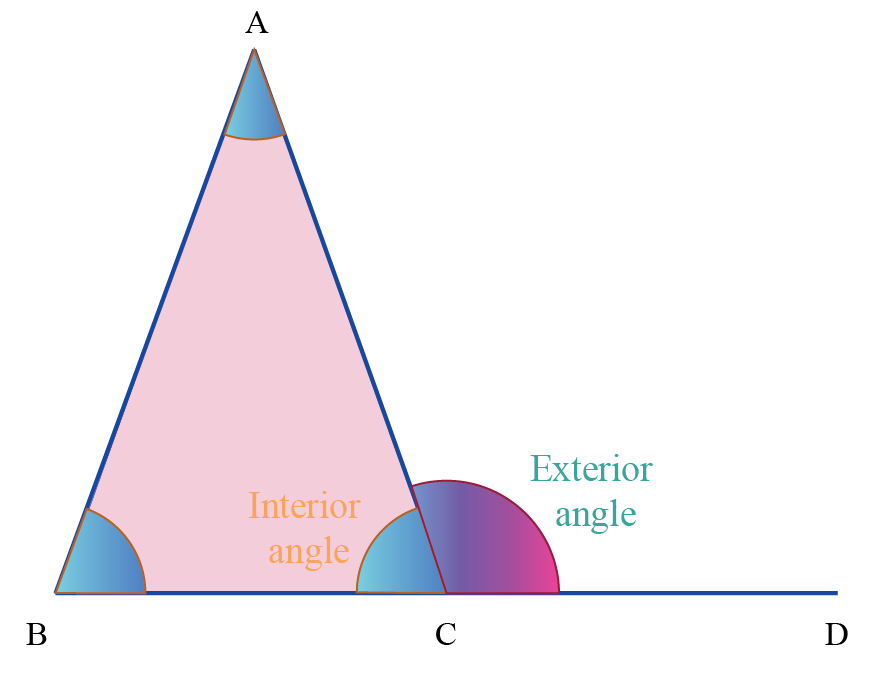## Complementary and Supplementary Angles

### Complementary Angles

When the sum of two angles is $${90^\circ}$$, the angles are called complementary angles.

Each angle is called the complement of the other angle.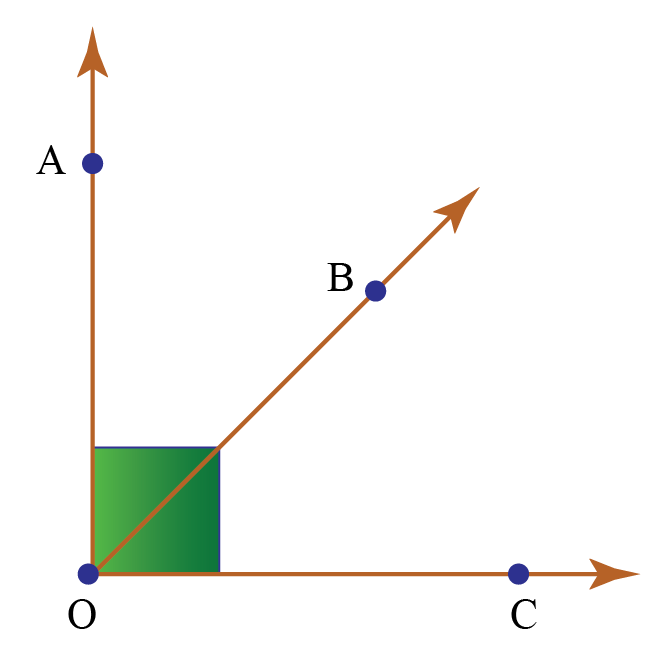$${\angle AOB}$$ + $${\angle BOC}$$ = $${90^\circ}$$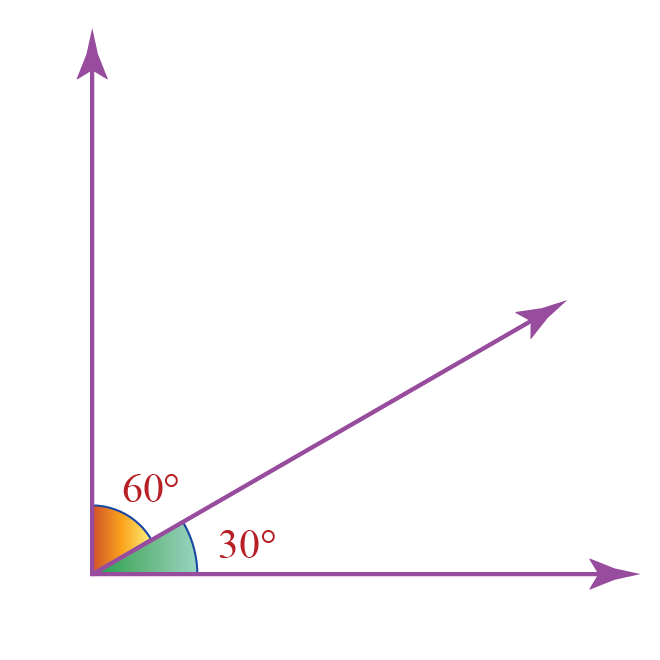$${60^\circ}$$ + $${30^\circ}$$ = $${90^\circ}$$

The above angles are complementary angles.

### Supplementary Angles

When the sum of two angles is $${180^\circ}$$, the angles are called supplementary angles.

Each angle is called the supplement of the other angle.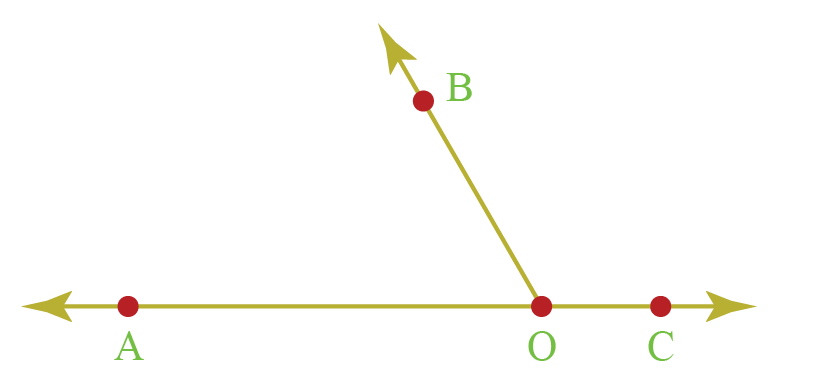$${\angle AOB}$$ + $${\angle BOC}$$ = $${180^\circ}$$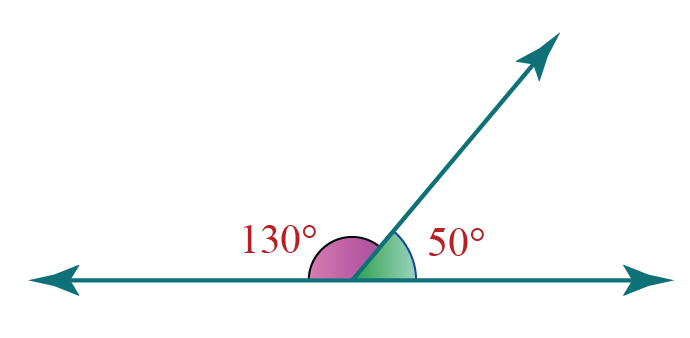$${130^\circ}$$ + $${50^\circ}$$ = $${180^\circ}$$

The above angles are supplementary angles

## Some more types of Angles

Any two angles that share-

• a common ray or side
• a common vertex
• and whose interiors do not overlap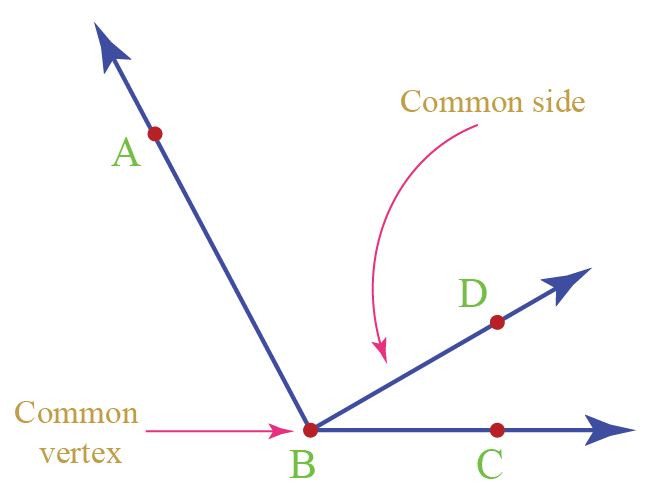Interiors of $${\angle ABD}$$ and $${\angle CBD}$$ don’t overlap and hence they are adjacent angles.

### Vertically Opposite Angles

The angles opposite to each other when two lines cross are called vertically opposite angles. They are always equal.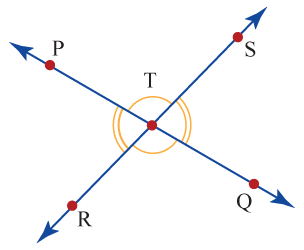Example: $${\angle PTS}$$ and $${\angle RTQ}$$ are vertically opposite angles.

## What is Transversal?

Consider two lines AB and CD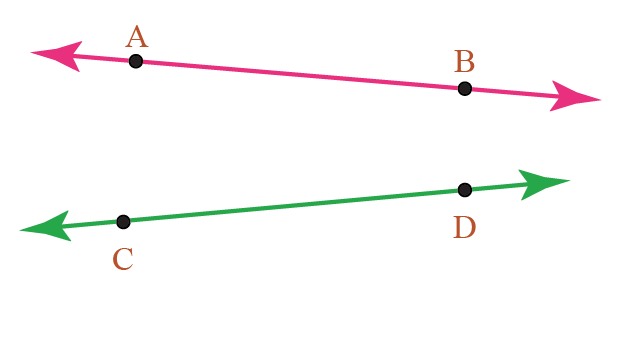Let line segment XY be the line that intersects these two lines at two distinct points, P and Q.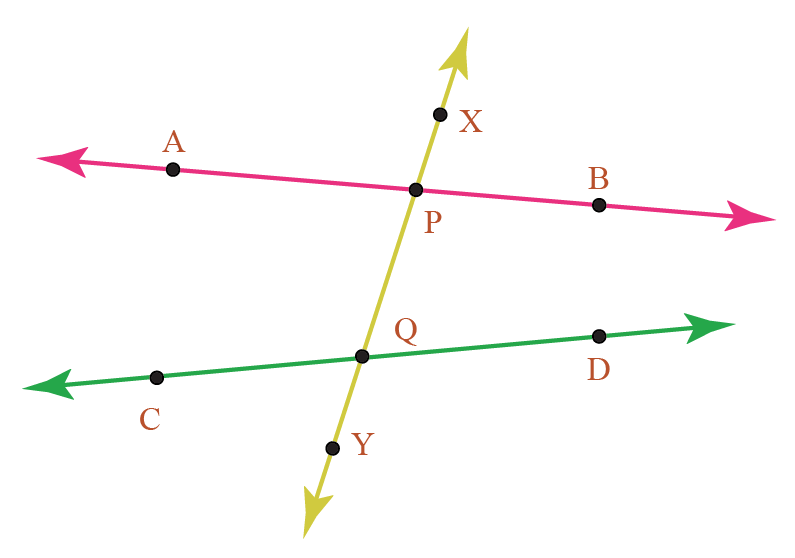A line that intersects two other lines at two distinct points is known as the transversal to the lines.

In the above image, XY is the transversal. When a transversal crosses a pair of parallel lines, you end up with different types of angles.

Let's discuss these angles.

### Corresponding Angles

Corresponding angles are the angles that:

• have different vertices
• lie on the same side of the transversal, and lie above (or below) the lines
• They are always equal.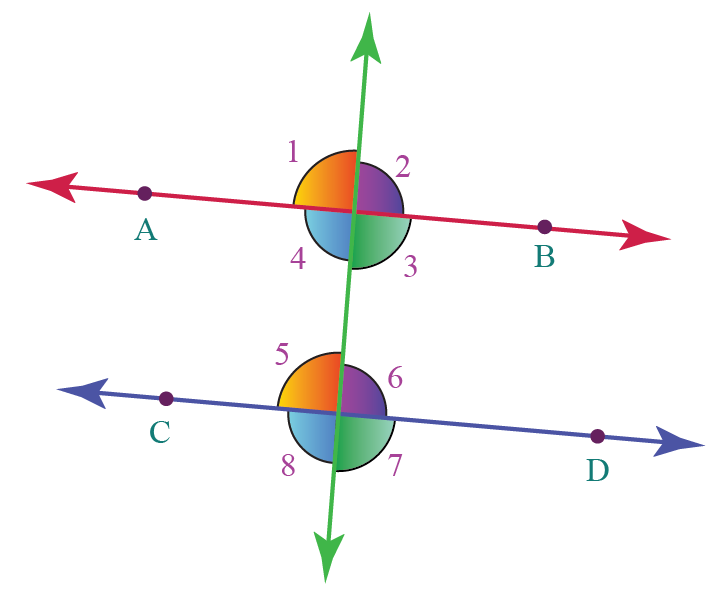When a transversal intersects two parallel lines, the corresponding angles formed are always equal.

In the above figure, $${\angle 1}$$ & $${\angle 5}$$, $${\angle 2}$$ & $${\angle 6}$$, $${\angle 4}$$ & $${\angle 8}$$, $${\angle 3}$$ & $${\angle 7}$$ are all pairs of corresponding angles.

### Alternate Interior Angles

Alternate-interior angles are those angles that:

• have different vertices
• lie on the alternate sides of the transversal
• lie between the interior of the two lines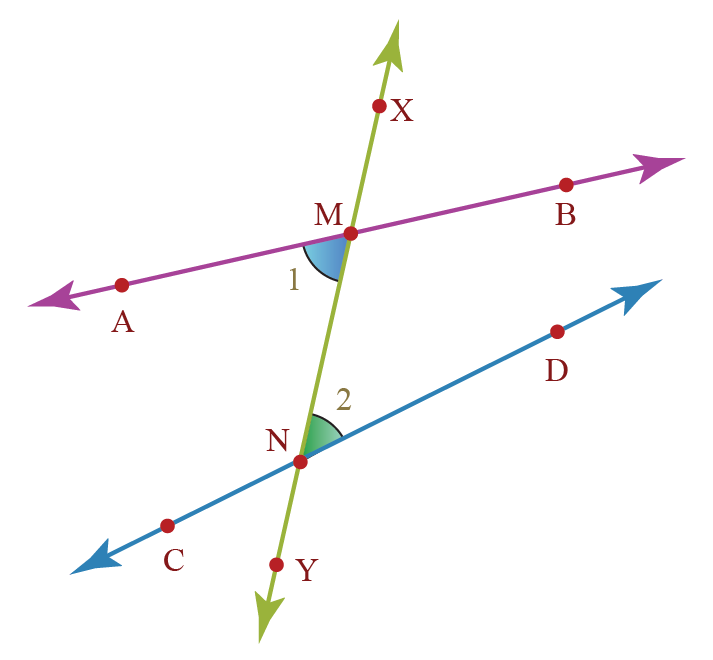When a transversal intersects two parallel lines, the alternate interior angles formed are always equal.

Here, $${\angle 1}$$ and $${\angle 2}$$ are the alternate interior angles.

### Alternate Exterior Angles

Alternate-exterior angles are those angles that:

• have different vertices
• lie on the alternate sides of the transversal
• are exterior to the lines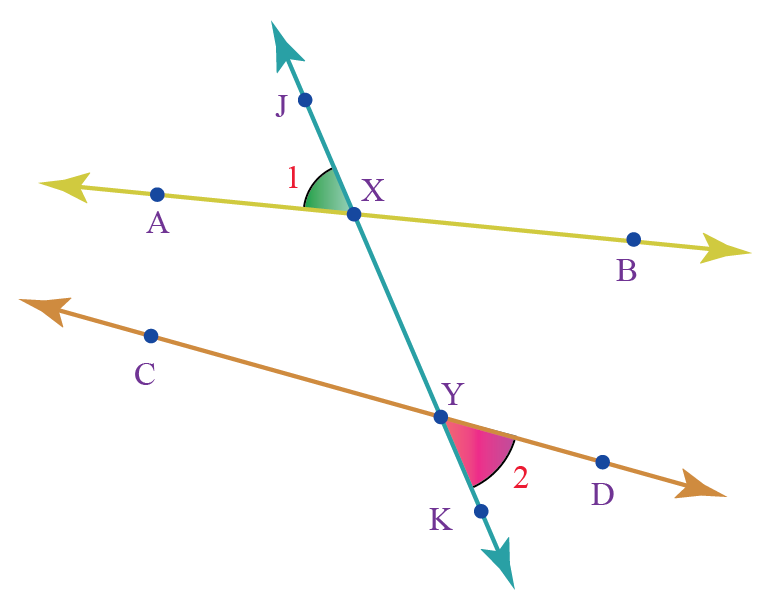When a transversal intersects two parallel lines, alternate exterior angles formed are always equal.

Here, $${\angle 1}$$ & $${\angle 2}$$ have different vertices.

They lie on the opposite side of the transversal.

Therefore, $${\angle 1}$$ & $${\angle 2}$$ are alternate exterior angles.

### Co-interior Angles

Co-interior angles are those angles that:

• have different vertices
• lie between two lines
• and are on the same side of the transversal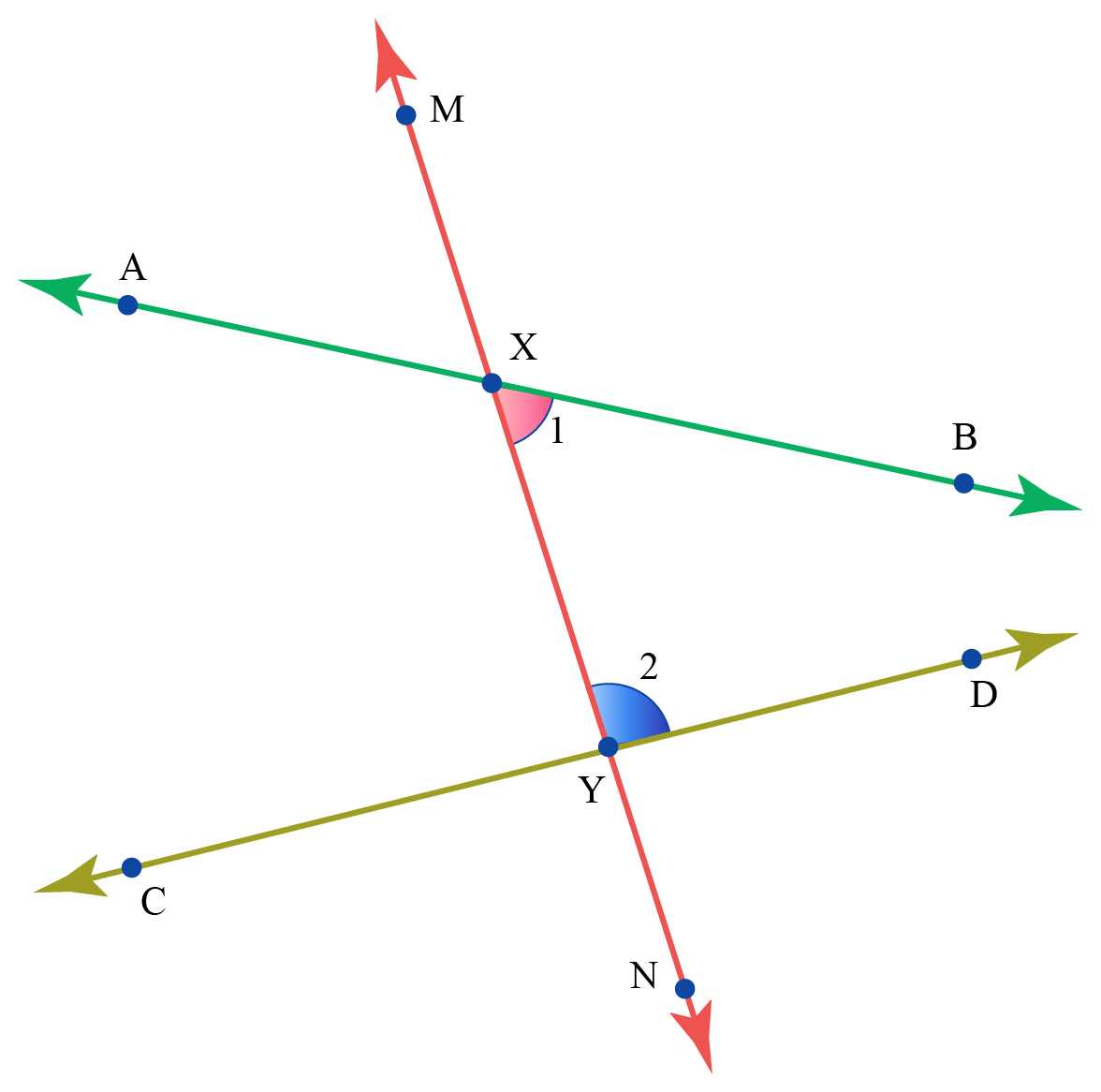When a transversal intersects two parallel lines, the co-interior angles are always supplementary.

In the above image, $${\angle 1}$$ & $${\angle 2}$$ have different vertices.

They lie on the same side of the transversal.

$${\angle 1}$$ & $${\angle 2}$$ are angles interior to the lines AB and CD respectively.

Therefore, $${\angle 1}$$ and $${\angle 2}$$ are co-interior angles and they are supplementary.

## Exterior Angle Property of a Triangle

An exterior angle of a triangle is always equal to the sum of the opposite interior angles.

Consider one of the exterior angles, $${\angle 4}$$ of △PQR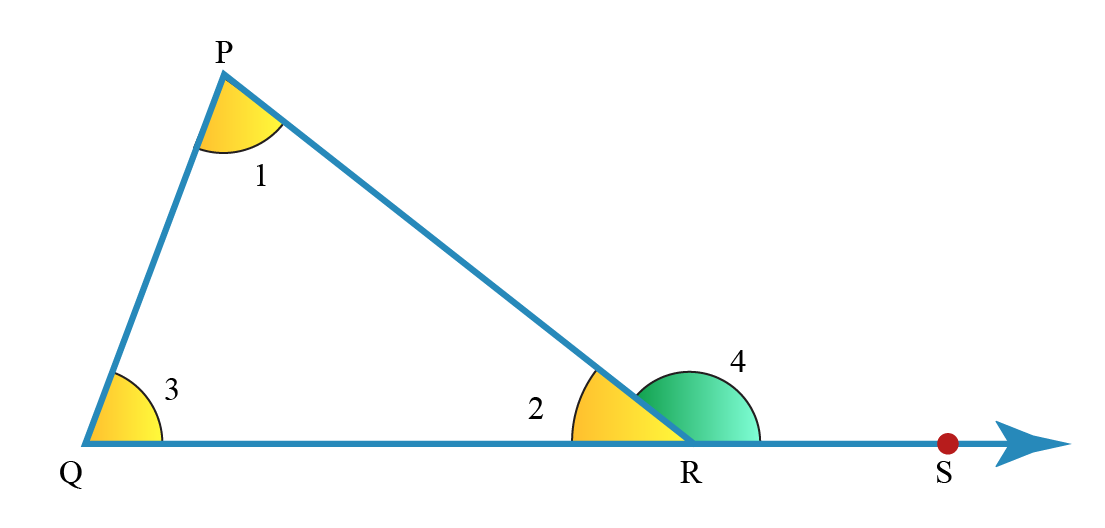In △PQR, by the angle sum property,

\begin{array}{l}
\angle 1+\angle 2+\angle 3=180^{\circ} \\
\angle 2+\angle 4=180^{\circ} \\
\angle 1+\angle 3=180^{\circ}-\angle 2\\
\angle 4=180^{\circ}-\angle 2
\end{array}

The sum obtained in both cases is equal, $${\angle 1}$$ + $${\angle 3}$$ = $${\angle 4}$$

In any triangle, an exterior angle is always equal to the sum of its interior opposite angles.

This is the exterior angle property of triangles.

## Angle types based on Rotation

Based on the direction of measurement or the direction of rotation, angles can be of two types:

1. Positive Angles
2. Negative Angles

### Positive Angles

An angle generated by the counterclockwise direction is a positive angle.

From the origin, if an angle is drawn in the $$(+\textit {x} ,+\textit {y})$$ plane, it forms a positive angle.

### Negative Angles

Negative angles are those angles which are measured in a clockwise direction from the base.

From the origin, if an angle is drawn towards the $$(-\textit {x} ,-\textit {y})$$ plane, it forms a negative angle.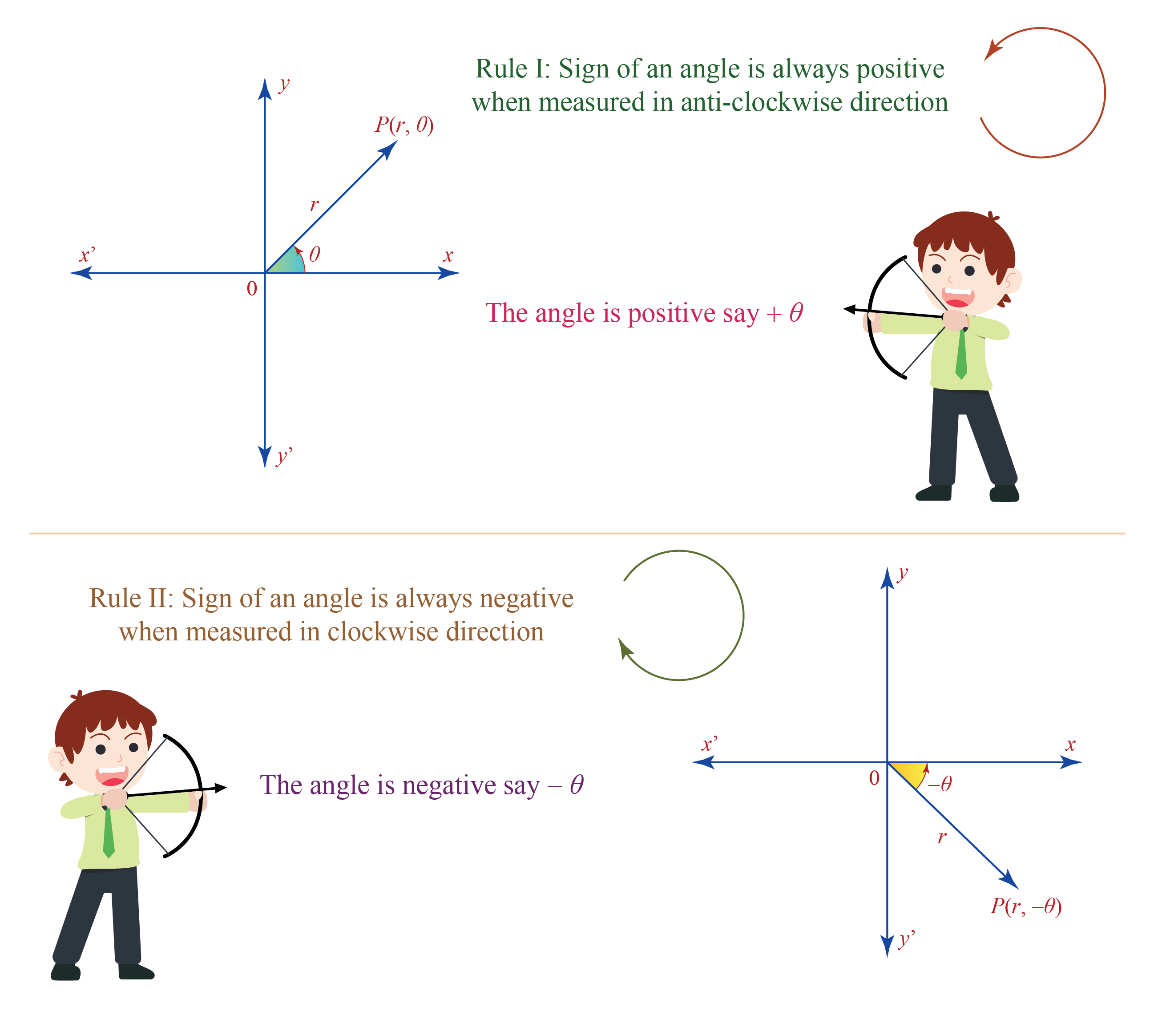Angles Worksheets
Angles Worksheets
Worksheet on Angles
Geometry
grade 9 | Questions Set 1
Geometry
Geometry
grade 9 | Questions Set 2
Geometry